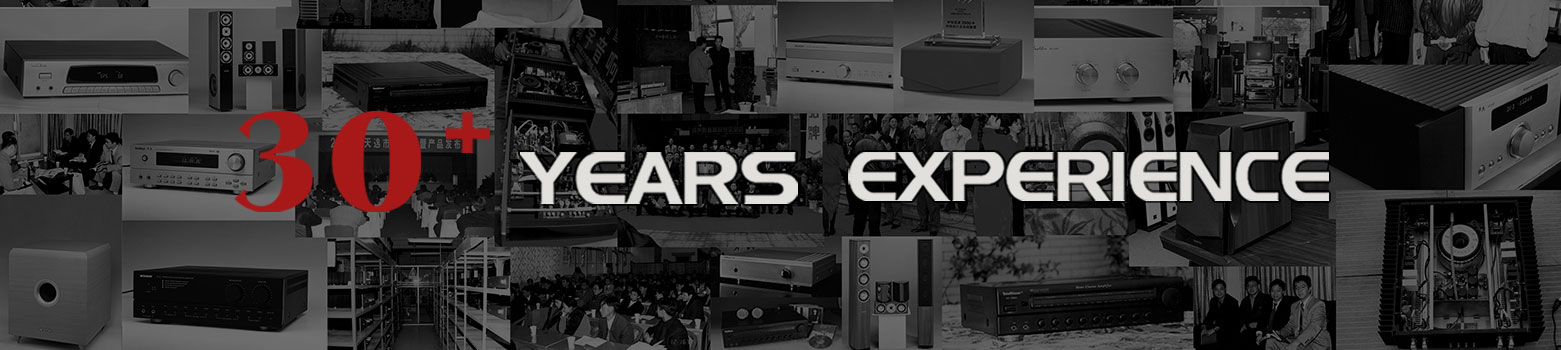What is the maximum sound pressure level? Is bigger better? Can sound pressure levels be superimposed directly? Mar 07 , 2023

What is the maximum sound pressure level? Is bigger better? Can sound pressure levels be superimposed directly?

1. What is the sound pressure level (maximum sound pressure level)?

The sound pressure level is a quantitative level that people divide for the convenience of distinguishing the strength of the sound, and is used to indicate the strength of the sound. The maximum sound pressure level is an important indicator of the microphone parameters. It refers to the measurement of the maximum sound pressure level that the microphone can withstand up to 0.5 total harmonic distortion, generally expressed in dB. The higher the value, the better.

The maximum input sound pressure level of the microphone is above 130dB, which is a very good microphone.

2. The most commonly used unit for sound pressure: decibels

Decibel is the unit of sound pressure level (symbol: db). For every doubling of sound pressure, the sound pressure level increases by 6 decibels.

Performance of sound intensity under various levels of sound pressure:

• 1 decibel is the sound that human ears can just hear!

Generally speaking, the sound below 20 decibels is considered quiet, and of course, generally speaking, the sound below 15 decibels can be regarded as "dead silence".

•  20-40 decibels, which is about whispering in the ears of a couple.
•  40-60 decibels, which belongs to our normal conversation sound.
•  Above 60 decibels, it belongs to the noisy range.
•  70 decibels, we can think it is very loud, and it starts to damage the hearing nerve.
•  Above 90 decibels, it will cause hearing loss.
•  In a space of 100-120 decibels, if there is no accident, humans will be temporarily deaf (deaf) in one minute. Among them, the noise of the car is between 80-100 decibels. Taking a car with a noise of 90 decibels as an example, you can still hear the noise of 81 decibels at a distance of 100 meters (the above standards will vary due to the difference in the environment, and are not absolute. value).

3. Theoretical calculation method of sound pressure level

Sound pressure level, the base 10 logarithm of the ratio of a given sound pressure to a reference sound pressure multiplied by 20, expressed in decibels. The sound pressure level and sound power level have the following relationship: Lp=Lw-10lg (4πr2), in the formula, r is the distance from the sound source to the test point.

For a specific point source, Lw is a constant value. When (4πr2)=1, that is, when r=0.282m, the sound pressure level is numerically equal to the sound power level; when (4πr2)<1, that is, when r<0.282m, the sound pressure level is greater than the sound power level, and vice versa The sound pressure level is less than the sound power level. When the noise is expressed as sound pressure level, the distance from the test point to the sound source shall be given.

The sound pressure level is represented by the symbol SPL, which is defined as the common logarithm of the ratio of the effective value of the sound pressure to be measured p(e) to the reference sound pressure p(ref), and then multiplied by 20, that is: SPL=20Log(10) [p(e)/p(ref)] in decibels.

In the air, the reference sound pressure p(ref) is generally taken as 2*10E-5 Pa. This value is the sound pressure value at which the normal human ear can just perceive the existence of a 1 kHz sound, that is, the possible sound pressure of a 1 kHz sound. Hearing threshold sound pressure. Generally speaking, below this sound pressure value, the human ear can no longer detect the existence of this sound. Obviously, the sound pressure level of the audible threshold sound pressure is zero decibels.

4. Can the sound pressure level be superimposed directly?

In actual work, we often encounter the problem of superposition of sound pressure levels when two or more speakers work together. So, can the sound pressure levels be directly superimposed? the answer is negative! The addition of two equal sound pressure levels only increases by 3dB instead of doubling the original.

Categories
Latest blog
Tags